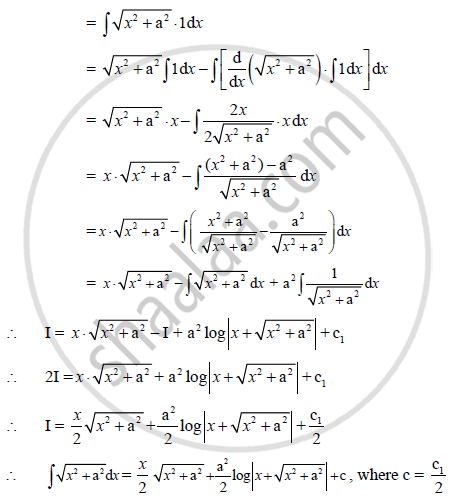HSC Arts 12th Board ExamMaharashtra State Board
Share

# Prove that Integral Sqrt(A^2 +X^2)Dx = X/2 Sqrt(A62 + X^2) + A^2/2 Log |X + Sqrt(X^2 + A^2)| + C - HSC Arts 12th Board Exam - Mathematics and Statistics

ConceptMethods of Integration - Integration Using Partial Fractions

#### Question

Prove that:

int sqrt(a^2 +x^2)dx = x/2 sqrt(a^2 + x^2) + a^2/2 log |x + sqrt(x^2 + a^2)| + c

#### Solution

Let I = int sqrt(x^2 + a^2)dxIs there an error in this question or solution?

#### APPEARS IN

2012-2013 (October) (with solutions)
Question 6.2.1 | 4.00 marks

#### Video TutorialsVIEW ALL 

Solution Prove that Integral Sqrt(A^2 +X^2)Dx = X/2 Sqrt(A62 + X^2) + A^2/2 Log |X + Sqrt(X^2 + A^2)| + C Concept: Methods of Integration - Integration Using Partial Fractions.
S